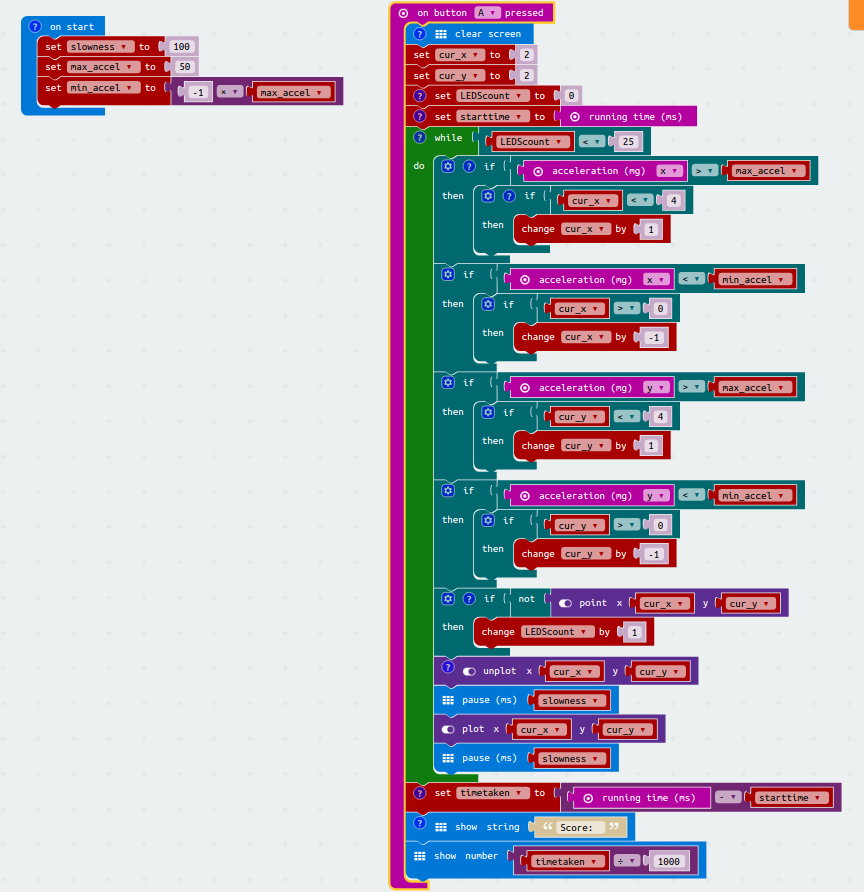# Fill It Up

You are going to make a game where the object is to light all the LEDs on the Micro:bit in the fastest possible time. One LED will flash and you will tilt the Micro:bit to roll the flashing LED around the screen. Every time it rolls to a new LED the new LED will light it up.

All you have to do is light up all 25 LEDs as fast as possible. Easy?

## Step1: Rolling an LED around the screen

We're going to use the LED screen coordinate system to control the movement of the LED. The screen has two coordinates: X, running from left to right and Y running from top to bottom. Each starts at 0 so the top left hand corner is X = 0 and Y=0 and the bottom right hand corner is X=4 and Y=4

Let's set an LED in the middle of the screen. This is where X=2 and Y=2. Since we will be changing these values we need to create 2 variables, let's call them 'cur_x' and 'cur_y'.
('cur' is short for 'current' and is often used in variable names to indicate that this is the current value of the variable but it may change)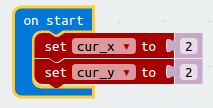That doesn't do much so let's light up the LED at that point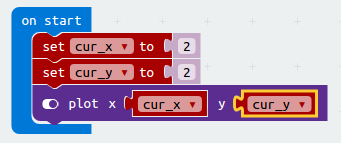We can use the accelerometer function of the Micro:bit to detect when the Micro:bit is being tilted. To start with we will just detect movement along the X-axis and light up the next LED when we detect a tilt

We'll need to do this in a Forever loop. Every time the Micro:bit is tilted to the right the X acceleration will become greater than 0 and so in that case we will increase the value of cur_x so that the next LED to the right lights up. Similarly if the Micro:bit is tilted to the left we need to decrease cur_x so the LED to the left is lit up.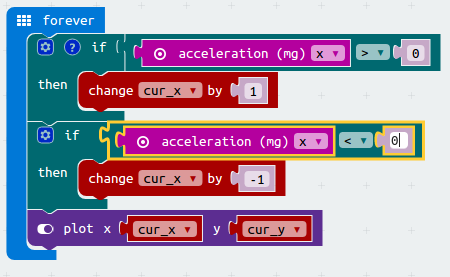Try it. It's very sensitive and hard to get just the next LED lit up. Slow it down by adding a pause statement (of say 100ms) after the plot statement in the forever loop

We can do the same thing for moving LEDs up and down, so let's add similar code for the Y axis - up and down movement## Step2:Fixing the bug!

Can you roll the LED round the screen. I suspect you are probably having difficulty and sometimes the LED just doesn't seem to be moving.

#### STOP - Can you think why?

Remember what we said earlier that the screen goes from X=0, Y=0 in the top left corner to X=4, Y=4 at the bottom right corner.

Got it yet? Whenever we detect any acceleration we increase or decrease the value of cur_x or cur_y. So cur_x or cur_y may have values bigger than 4 or less than 0. When we use a plot statement with values outside the screen such as "plot x -2 y 7" then nothing gets plotted. So we only see LEDs being plotted when cur_x and cur_y have values between 0 and 4 inclusive.

To fix this we need to put some test into our code so that if cur_x or cur_y are already 0 or 4 we don't change them and make them go out of range.

Here's the code to stop cur_x being >4Can you write the code to make sure cur_x and cur_y are always within the range 0 to 4?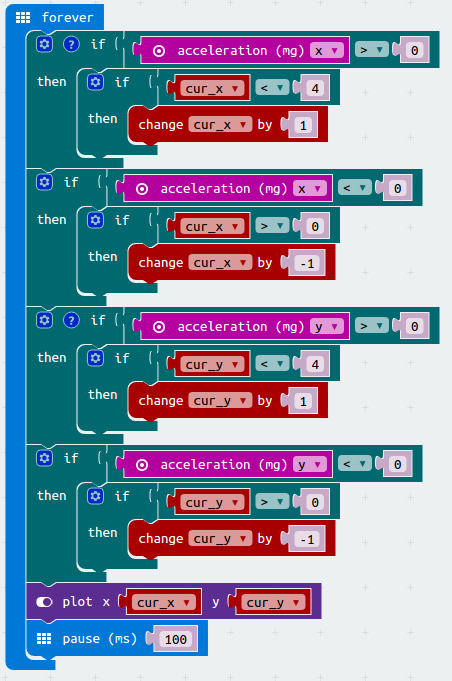Well, that might be working, it's hard to tell because we can't see where we are. Let's add an unplot so that we can see that we are just moving around the LEDs correctly.That's great - we can move the LED around, just tilting the Micro:bit and it always has a LED lit. However, before we added that unplot we almost had a complete game, the square of LEDs was filling up nicely.

What's the difference? Well, now the last thing in our Forever loop is an unplot which turns the LED off, so when we move to a new position the previous LED is turned off. Previously our loop ended with a plot and so the LEDs stayed on. So having the plot at the end of the loop is good. What do you think will happen if we do the unplot first and then the plot?

Let's try it, adding a delayWow - that works! The screen fills up nicely and the current position of the LED we tilt is shown by flashing - perfect. We have a game.

Almost!

## Step 3: Checking for Game End

How do you think we know when the game ends?

That's right, all 25 LEDs are lit up. So we need a variable that counts every time we light up an LED and when it reaches 25 we know we have finished.

What happens when we move back over an LED that is already lit? We definitely don't want to count it again. So we need to make sure we only count LEDs that are unlit before we tilt onto them for the first time whicxh is what makes them become lit

So first of all we need a variable, and it is going to start off with the value 0 so no LEDs are lit. We can put this in our On Start code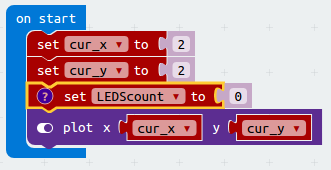Now after we have just moved (i.e. all those "if acceleration" statements) we need to check if the point we have moved to is unlit and if it is we can add one to our countOK so now we know how many LEDs are lit up and we know we want the game to end when 25 LEDs are lit. How are we going to use this? Also can we make the game start again when we've finished?

Instead of it starting the game automatically we'll make it only start when button A is pressed. And instead of a Forever loop we'll code a While loop that continues for as long as there are LEDs still needing lighting - that is as long as LEDScount is less than 25.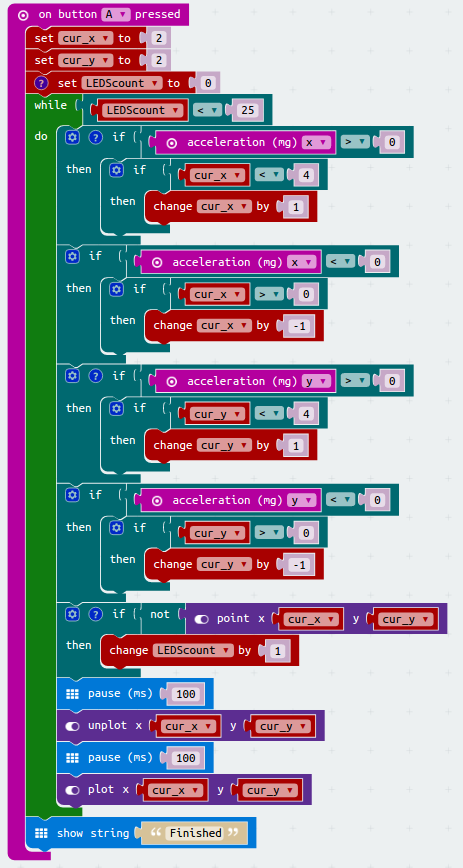That works. As some as the screen fills up we put put the message "Finished"

## Step 4: Adding a timer

Great, now if we could just replace that finished message with a message that says how long it took to fill the screen we would have a good game.

The Micro:bit has a clock (runninngtime) that says how long it has been running (in milliseconds). So we can tell how long we have taken to complete the game by looking at the time when we start the game and the time when we end the game and subtracting.

So at the beginning of the On Button A block before the While loop let's add another variable to record the starttime.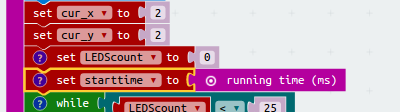And at the end of the game we can work out the time taken in milliseconds. If we then divide this by 1000 we get seconds which is better to tell the user. We also need to report this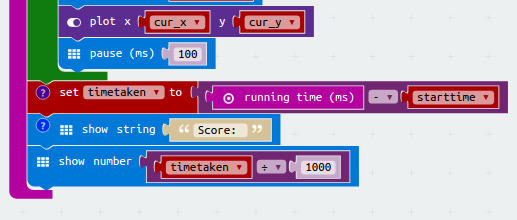That's it

Almost!

If we press button A we get a new game but the screen is full up so we can't play. Simple to fix, we just need to a a Clear Screen instruction everytime we start the game.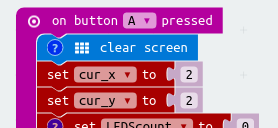## Step 5: Making it easier

The game is very difficult to control. That is because whenever we notice that the Micro:bit's acceleration is not 0 we move the LED. This makes the game very 'twitchy' - it would be a lot less sensitive if we only moved the LED if the accereration was greater than a value bigger than 0 - the bigger the value we choose, the less sensitive the game becomes. Let's try a value of 50 and so we want to replace all of the code that says `if acceleration(mg)x > 0` with `if acceleration(mg)x > 50` and the same for `acceleration(mg)y`. We also want to replace `if acceleration(mg)x < 0` with `if acceleration(mg)x < -50` and again for `acceleration(mg)y`.

We can easily make these changes, but what happens if we find it is still too sensitive and we want to try a sensitivity of 100 rather than 50? We have to changes the code in lots of places. Whenever we change code in several places there is always a chance we will miss one and so introduce a bug into our code. How can we avoid this?

It is good coding practice to use a variable such as `max_accel` which we set once and once only in our On Start code and then do tests such as `if acceleration(mg)x > max_accel`. Now if we want to make a change we just need to change the single line of code where we give `max_accel` a value and everywhere in the code will use the new value. No chance of missing anywhere and introducing a bug.

So we will set `max_accel` in oue On Start block and also to allow for the less than tests we will define another variable min_accel as -1*max_accel (i.e. if we set max_accel to 50 then min_accel will be -50, just what we need for the `if acceleration(mg)x < ...` tests.

Another way of tweaking the speed of the code is to adjust the delay every time we plot and unplot the flashing LED. Currently we have this as a fixed 100 milli-second delay but we might want to explore changing this to make the game easier or harder so let's also replace those `pause(ms) 100` lines to test a variable called slowness.

Here's the On Start code:#### Complete Code